# Thresholding Image Stacks

## Stacks of Images

`50.tif` is a TIFF file which is a stack of 50 images of a bit of a cell taken over a short space of time (i.e. it’s a video).

``````img <- ijtiff::read_tif(system.file("extdata", "50.tif",
package = "autothresholdr"
))
#> Reading 50.tif: an 8-bit, 100x100 pixel image of unsigned
#> integer type. Reading 1 channel and 50 frames . . .
#>  Done.
dim(img)
#>  100 100   1  50``````

Let’s display the first 3 frames: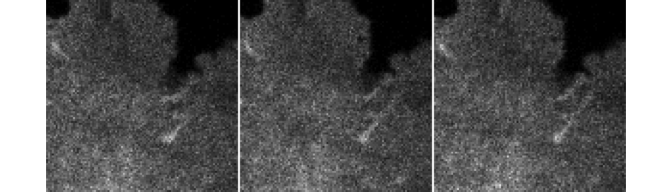and the last 3 frames: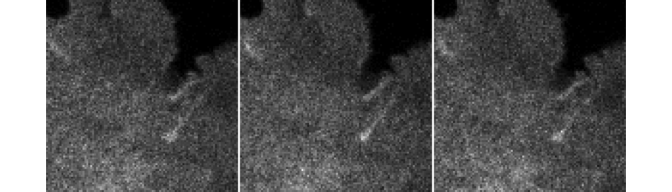You’ll notice that these images are almost identical. That’s because they’re images of the same area taken very quickly one after another. There are two ways to threshold an image stack like this. One is the naiive way: find a threshold based on every pixel in the stack, then pixels below the threshold are excluded (set to `NA`). Let’s try that:

``````library(autothresholdr)
attr(naiively_threshed_img, "thresh") # The threshold chosen by "Triangle" is 4
#>  4
#> attr(,"ignore_black")
#>  FALSE
#> attr(,"ignore_white")
#>  FALSE
#> attr(,"ignore_na")
#>  FALSE
#> attr(,"autothresh_method")
#>  "Triangle"
#> attr(,"class")
#>  "th"      "integer"``````

Now let’s display the first 3 frames and the last 3 frames: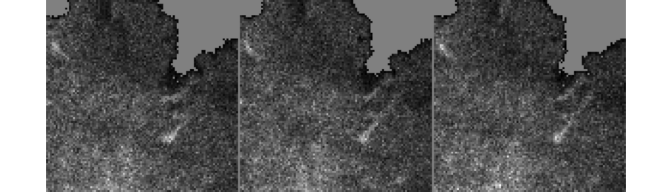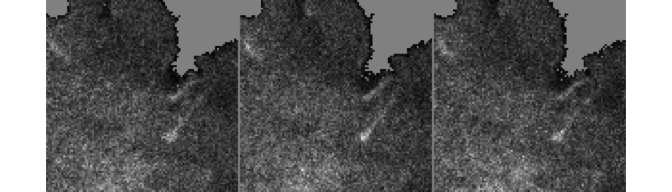If you look closely, you can see that the threshold mask is (slightly) different for different frames. Let’s highlight the pixels which are sometimes thresholded away, sometimes not: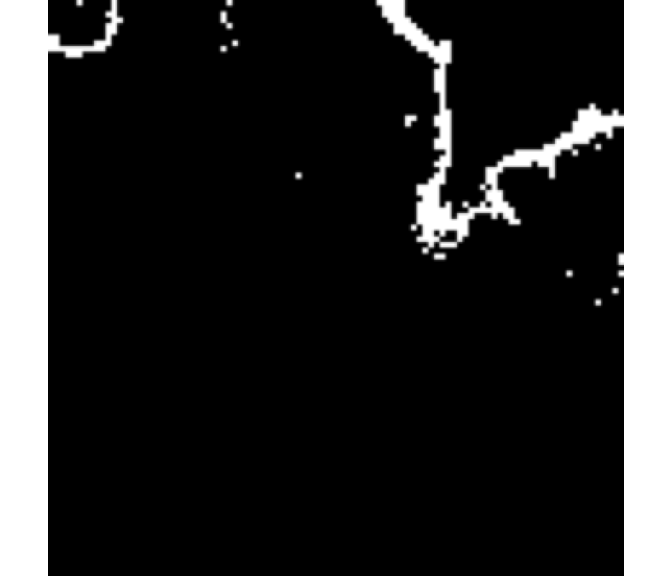So (unsurprisingly), it seems that around the edges of the cell (where the signal from the cell is more feint), the pixels are sometimes thresholded away, sometimes not. There are also some seemingly random pixels within the cell which are sometimes thresholded away, sometimes not.

Now, given that you know that the cell is more or less stationary and you want the threshold to get rid of the non-cell bits and keep the cell bits, its reasonable to assert that the mask should be the same for every frame. It’s possible to apply the same mask to every frame, and to compute this mask, it makes sense to incorporate information from all of the frames. This is what `mean_stack_thresh()` and `med_stack_thresh()` do. `mean_stack_thresh()` computes the mask based on the mean of all of the frames (gotten by calculating the mean intensity of the stack at each pixel position). `med_stack_thresh()` uses the median instead of the mean. They’re both very similar. If you don’t know which one to use, just use `mean_stack_thresh()`.

Let’s visualize them both:

``````ijtiff::display(mean_stack_thresh(img, "tri"))
#> Only the first frame of the image stack is displayed.
#> To display all frames use 'all = TRUE'.``````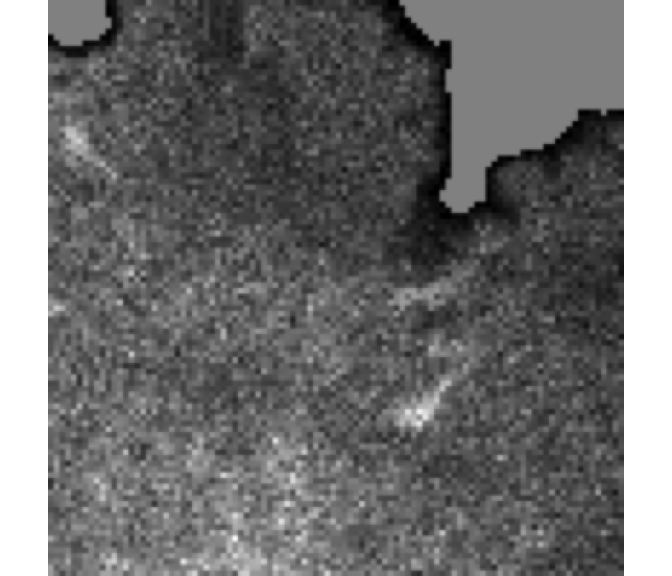``````ijtiff::display(med_stack_thresh(img, "tri"))
#> Only the first frame of the image stack is displayed.
#> To display all frames use 'all = TRUE'.``````